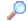Search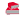Directory

Desktop Functions:

Smart Device Functions: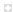Glossary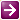Show Recent ChangesSubscribe (RSS)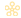Misc. Pages
GetKeyState (user32)

Summary
The GetKeyState function retrieves the status of the specified virtual key. The status specifies whether the key is up, down, or toggled (on, off—alternating each time the key is pressed).

#### C# Signature:

[DllImport("USER32.dll")]
static extern short GetKeyState(VirtualKeyStates nVirtKey);

#### VB.NET Signature

<DllImport("user32.dll", SetLastError := True, CharSet := CharSet.Unicode)> _
Private Function GetKeyState (ByVal nVirtKey As KeyStates) As Short
End Function

VK

#### Windows Input Simulator

An open source managed .NET wrapper written in C# is available on Codeplex at http://inputsimulator.codeplex.com/. It has all of these definitions complete with documented code comments and can be used to determine key states as well. Thanks to all the contributors at pinvoke.

#### Notes:

The following two constants are used in combination with the returned value to test the current state:

Private Const KEY_TOGGLED As Integer = &H1

Private Const KEY_PRESSED As Integer = &H8000

Here are some sample values for nVirtKey:

enum VirtualKeyStates : int
{
VK_LBUTTON       = 0x01,
VK_RBUTTON       = 0x02,
VK_CANCEL    = 0x03,
VK_MBUTTON       = 0x04,
//
VK_XBUTTON1      = 0x05,
VK_XBUTTON2      = 0x06,
//
VK_BACK      = 0x08,
VK_TAB       = 0x09,
//
VK_CLEAR     = 0x0C,
VK_RETURN    = 0x0D,
//
VK_SHIFT     = 0x10,
VK_CONTROL       = 0x11,
VK_PAUSE     = 0x13,
VK_CAPITAL       = 0x14,
//
VK_KANA      = 0x15,
VK_HANGEUL       = 0x15,  /* old name - should be here for compatibility */
VK_HANGUL    = 0x15,
VK_JUNJA     = 0x17,
VK_FINAL     = 0x18,
VK_HANJA     = 0x19,
VK_KANJI     = 0x19,
//
VK_ESCAPE    = 0x1B,
//
VK_CONVERT       = 0x1C,
VK_NONCONVERT    = 0x1D,
VK_ACCEPT    = 0x1E,
VK_MODECHANGE    = 0x1F,
//
VK_SPACE     = 0x20,
VK_PRIOR     = 0x21,
VK_NEXT      = 0x22,
VK_END       = 0x23,
VK_HOME      = 0x24,
VK_LEFT      = 0x25,
VK_UP        = 0x26,
VK_RIGHT     = 0x27,
VK_DOWN      = 0x28,
VK_SELECT    = 0x29,
VK_PRINT     = 0x2A,
VK_EXECUTE       = 0x2B,
VK_SNAPSHOT      = 0x2C,
VK_INSERT    = 0x2D,
VK_DELETE    = 0x2E,
VK_HELP      = 0x2F,
//
VK_LWIN      = 0x5B,
VK_RWIN      = 0x5C,
VK_APPS      = 0x5D,
//
VK_SLEEP     = 0x5F,
//
VK_MULTIPLY      = 0x6A,
VK_SEPARATOR     = 0x6C,
VK_SUBTRACT      = 0x6D,
VK_DECIMAL       = 0x6E,
VK_DIVIDE    = 0x6F,
VK_F1        = 0x70,
VK_F2        = 0x71,
VK_F3        = 0x72,
VK_F4        = 0x73,
VK_F5        = 0x74,
VK_F6        = 0x75,
VK_F7        = 0x76,
VK_F8        = 0x77,
VK_F9        = 0x78,
VK_F10       = 0x79,
VK_F11       = 0x7A,
VK_F12       = 0x7B,
VK_F13       = 0x7C,
VK_F14       = 0x7D,
VK_F15       = 0x7E,
VK_F16       = 0x7F,
VK_F17       = 0x80,
VK_F18       = 0x81,
VK_F19       = 0x82,
VK_F20       = 0x83,
VK_F21       = 0x84,
VK_F22       = 0x85,
VK_F23       = 0x86,
VK_F24       = 0x87,
//
VK_NUMLOCK       = 0x90,
VK_SCROLL    = 0x91,
//
VK_OEM_NEC_EQUAL = 0x92,   // '=' key on numpad
//
VK_OEM_FJ_JISHO  = 0x92,   // 'Dictionary' key
VK_OEM_FJ_MASSHOU= 0x93,   // 'Unregister word' key
VK_OEM_FJ_TOUROKU= 0x94,   // 'Register word' key
VK_OEM_FJ_LOYA   = 0x95,   // 'Left OYAYUBI' key
VK_OEM_FJ_ROYA   = 0x96,   // 'Right OYAYUBI' key
//
VK_LSHIFT    = 0xA0,
VK_RSHIFT    = 0xA1,
VK_LCONTROL      = 0xA2,
VK_RCONTROL      = 0xA3,
//
VK_BROWSER_BACK       = 0xA6,
VK_BROWSER_FORWARD    = 0xA7,
VK_BROWSER_REFRESH    = 0xA8,
VK_BROWSER_STOP       = 0xA9,
VK_BROWSER_SEARCH     = 0xAA,
VK_BROWSER_FAVORITES  = 0xAB,
VK_BROWSER_HOME       = 0xAC,
//
VK_VOLUME_DOWN    = 0xAE,
VK_VOLUME_UP      = 0xAF,
VK_MEDIA_NEXT_TRACK   = 0xB0,
VK_MEDIA_PREV_TRACK   = 0xB1,
VK_MEDIA_STOP     = 0xB2,
VK_MEDIA_PLAY_PAUSE   = 0xB3,
VK_LAUNCH_MAIL    = 0xB4,
VK_LAUNCH_MEDIA_SELECT= 0xB5,
VK_LAUNCH_APP1    = 0xB6,
VK_LAUNCH_APP2    = 0xB7,
//
VK_OEM_1     = 0xBA,   // ';:' for US
VK_OEM_PLUS      = 0xBB,   // '+' any country
VK_OEM_COMMA     = 0xBC,   // ',' any country
VK_OEM_MINUS     = 0xBD,   // '-' any country
VK_OEM_PERIOD    = 0xBE,   // '.' any country
VK_OEM_2     = 0xBF,   // '/?' for US
VK_OEM_3     = 0xC0,   // '`~' for US
//
VK_OEM_4     = 0xDB,  //  '[{' for US
VK_OEM_5     = 0xDC,  //  '\|' for US
VK_OEM_6     = 0xDD,  //  ']}' for US
VK_OEM_7     = 0xDE,  //  ''"' for US
VK_OEM_8     = 0xDF,
//
VK_OEM_AX    = 0xE1,  //  'AX' key on Japanese AX kbd
VK_OEM_102       = 0xE2,  //  "<>" or "\|" on RT 102-key kbd.
VK_ICO_HELP      = 0xE3,  //  Help key on ICO
VK_ICO_00    = 0xE4,  //  00 key on ICO
//
VK_PROCESSKEY    = 0xE5,
//
VK_ICO_CLEAR     = 0xE6,
//
VK_PACKET    = 0xE7,
//
VK_OEM_RESET     = 0xE9,
VK_OEM_JUMP      = 0xEA,
VK_OEM_PA1       = 0xEB,
VK_OEM_PA2       = 0xEC,
VK_OEM_PA3       = 0xED,
VK_OEM_WSCTRL    = 0xEE,
VK_OEM_CUSEL     = 0xEF,
VK_OEM_ATTN      = 0xF0,
VK_OEM_FINISH    = 0xF1,
VK_OEM_COPY      = 0xF2,
VK_OEM_AUTO      = 0xF3,
VK_OEM_ENLW      = 0xF4,
VK_OEM_BACKTAB   = 0xF5,
//
VK_ATTN      = 0xF6,
VK_CRSEL     = 0xF7,
VK_EXSEL     = 0xF8,
VK_EREOF     = 0xF9,
VK_PLAY      = 0xFA,
VK_ZOOM      = 0xFB,
VK_NONAME    = 0xFC,
VK_PA1       = 0xFD,
VK_OEM_CLEAR     = 0xFE
}

#### Tips & Tricks:

The Enum Keys in System.Windows.Forms has the key code used for this function, so you don't need to define const. If casting in this manner, beware that the Keys.Shift member does not behave in the way that you would expect; you should use one of Keys.Menu, Keys.LMenu, Keys.RMenu instead. Return value will be 0 if off and 1 if on as a toggle and -127 if key held down.

Do not use (ByVal nVirtKey As Long) but (ByVal nVirtKey As Short), in VS2005, in fact, in the first case you will have a PInvoke error.

#### Sample Code:

This Sample use this API to create a new textbox to support overwrite mode, testing the state of insert key.

Imports System.Windows.Forms
Public Class MinhaNovaTextbox
Inherits System.Windows.Forms.TextBox

Dim bInserting As Boolean = True

Private Declare Function GetKeyState _
Lib "user32" (ByVal nVirtKey As Short) As Integer

Public Sub New()
MyBase.New()
bInserting = GetKeyState(Keys.Insert)
End Sub

Protected Overrides Sub OnKeyPress(ByVal e As System.Windows.Forms.KeyPressEventArgs)
bInserting = GetKeyState(Keys.Insert)
If Not bInserting Then
Me.SelectionLength = 1
End If
MyBase.OnKeyPress(e)
End Sub
End Class

This sample c# code returns if the mouse button is currently pressed.

private const int KEY_PRESSED = 0x8000;

public bool IsPressed(){

return Convert.ToBoolean(GetKeyState(VirtualKeyStates.VK_LBUTTON) & KEY_PRESSED);

}

#### Alternative Managed API:

You can get the state of the Control, Alt, and Shift keys using the static property Control.ModifierKeys.

Documentation
GetKeyState on MSDN

Do you have...

• helpful tips or sample code to share for using this API in managed code?
• corrections to the existing content?
• variations of the signature you want to share?
• additional languages you want to include?

Select "Edit This Page" on the right hand toolbar and edit it! Or add new pages containing supporting types needed for this API (structures, delegates, and more).Access PInvoke.net directly from VS:Terms of UseEdit This PageFind ReferencesShow Printable Version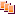Revisions Show changes